### Vector Calculus

Combining vectors together with calculus concepts creates new kinds of derivatives and integrals, many of which we'll explore through their use in the physical world.

To get a sense of what's to come, we'll use problems from fluid physics to introduce the surface integral, an important extension of the double integral of multivariable calculus.

No prior physics experience is required, however.

# Introducing Surface Integrals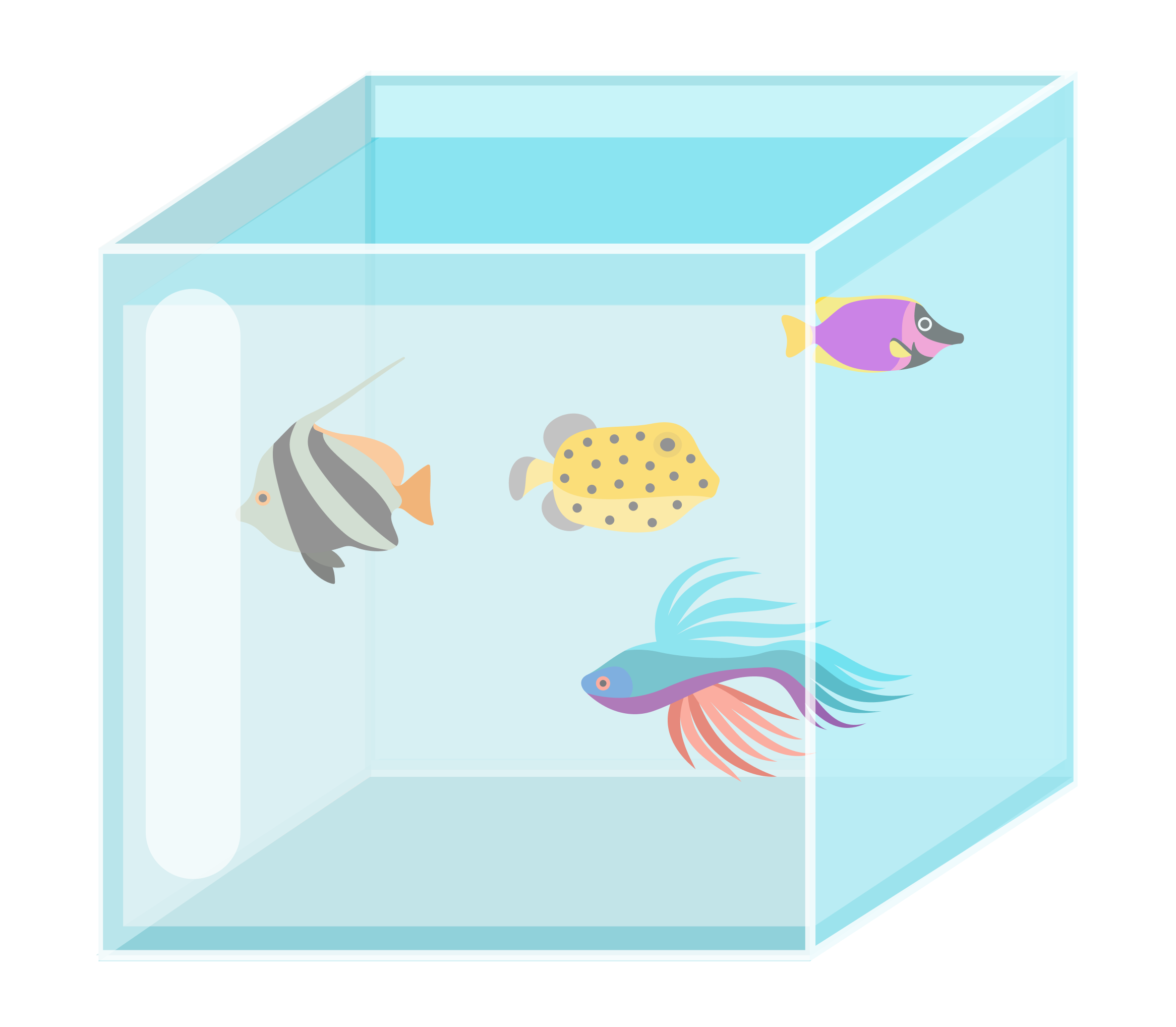Today's Arthur's first day apprenticing at Tanks For All The Fish, a business of building aquariums. No job is too big or too small, and no tank shape is too weird or too difficult.

His first task is to build a small cubic fish tank $l$ units on a side.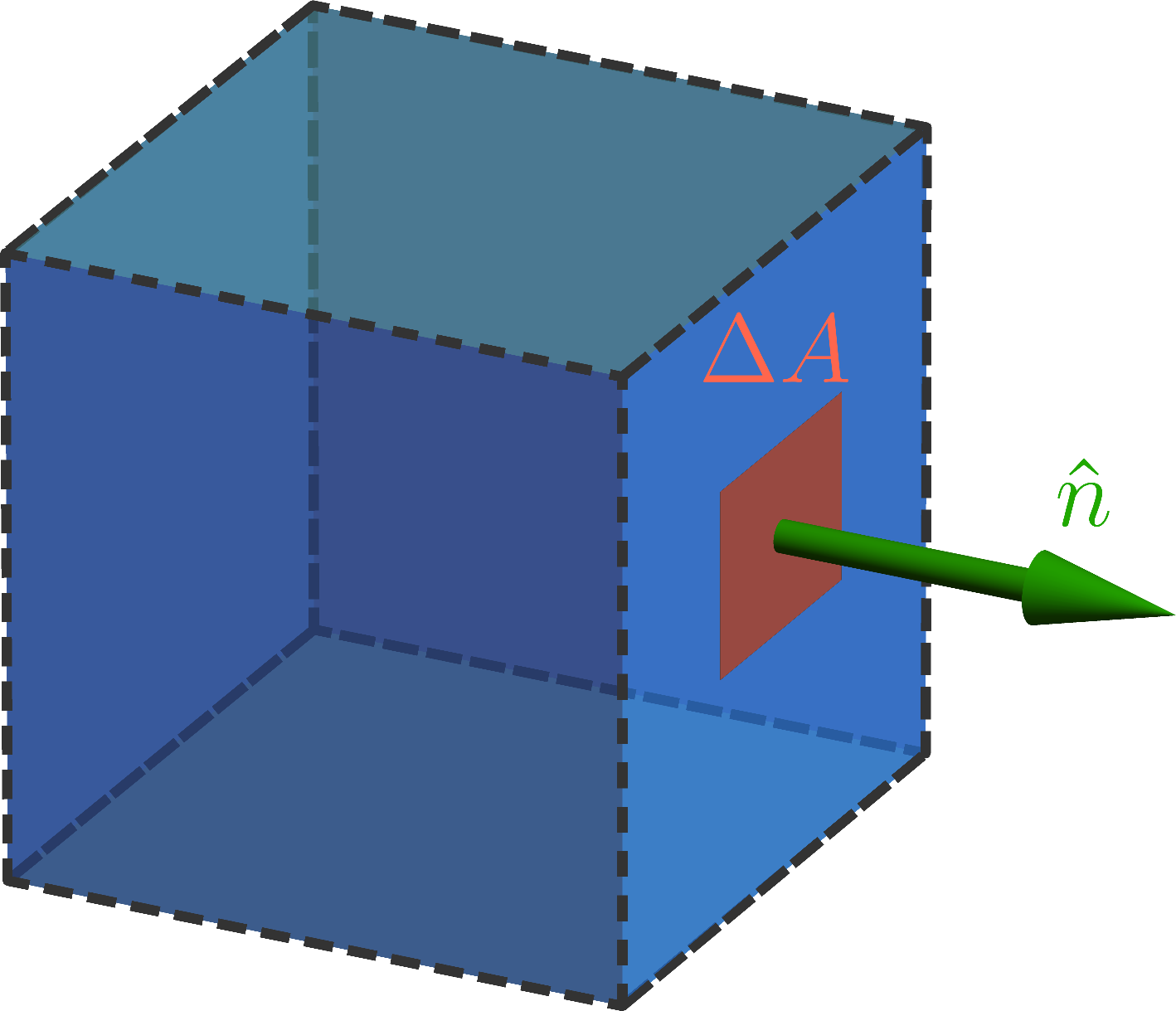According to his boss, the renowned Mr. D. Adams, the total force exerted on the container walls is an important quantity to keep in mind when choosing building materials.

The force on a small patch of area $\triangle A$ is given by the pressure $p_{_\text{fluid}}$ times the area, and is directed perpendicularly outward along a unit normal $\hat{n}.$

What should Arthur find as the total force vector on the bottom of the tank, assuming the pressure is constant there? Use the coordinate system displayed here: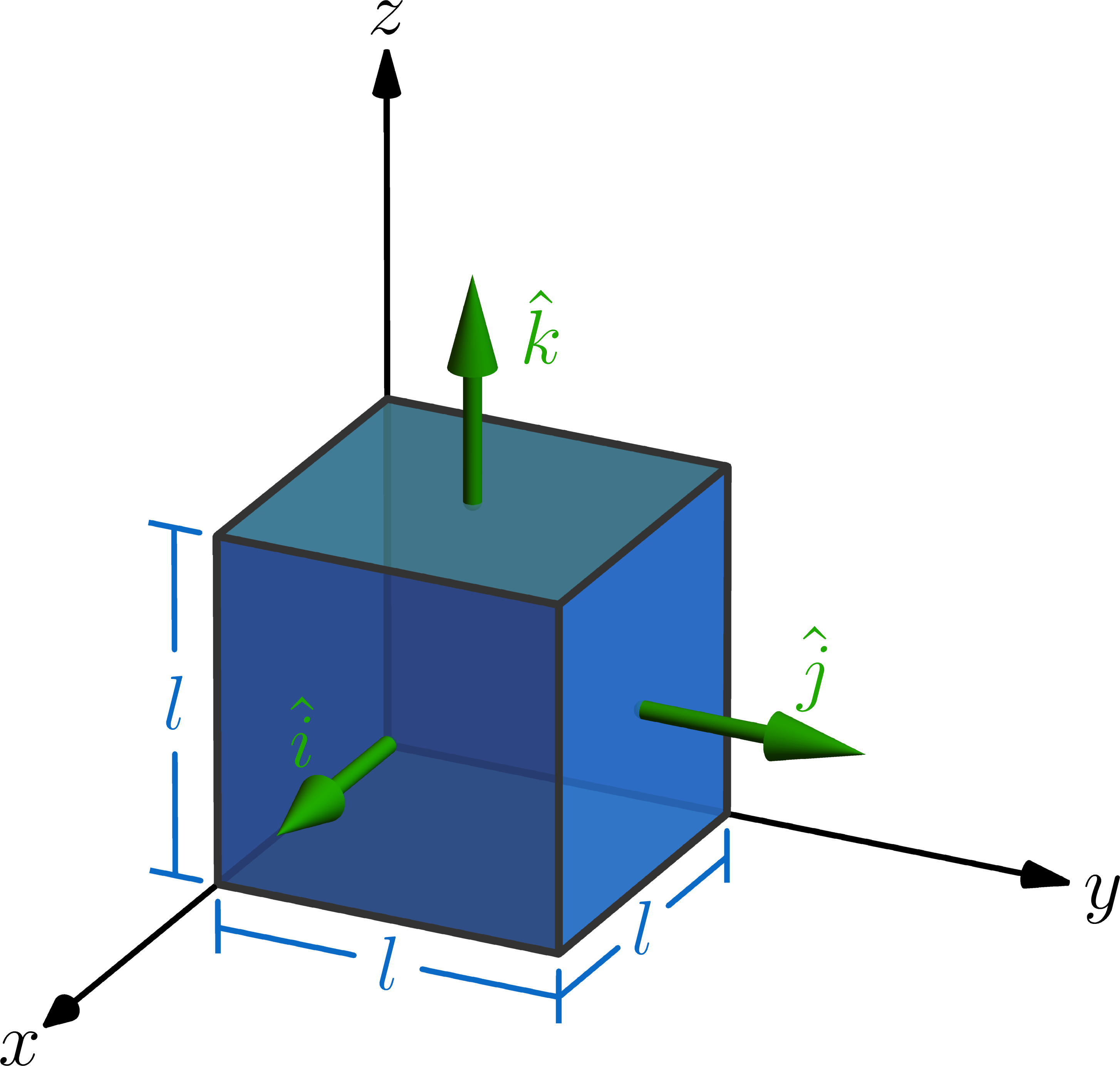# Introducing Surface Integrals

To find the total force vector on the bottom of the cubic aquarium tank, we assumed constant fluid pressure.

We got away with this only because the fluid is in hydrostatic equilibrium (i.e. it's not sloshing or moving around) and every point on the bottom of the tank is at the same depth below the water.

The visualization below demonstrates a very important rule in the aquarium building business:

The pressure on any small patch of the container wall is proportional to the depth below the surface, and the fluid force is directed perpendicularly outward.

The fluid the patch holds back exerts a total force (blue arrow). The $z$-axis is vertical, and the patch's center is located at $(a,b,c).$ Here $\theta$ measures the angle between the vector and the $xy$-plane while $\varphi$ controls the patch's orientation about the $z$-axis.

What conclusion can be drawn from this graph?

# Introducing Surface Integrals

Mr. Adams tasks Arthur with computing the total force vector on the side wall located at $x = l.$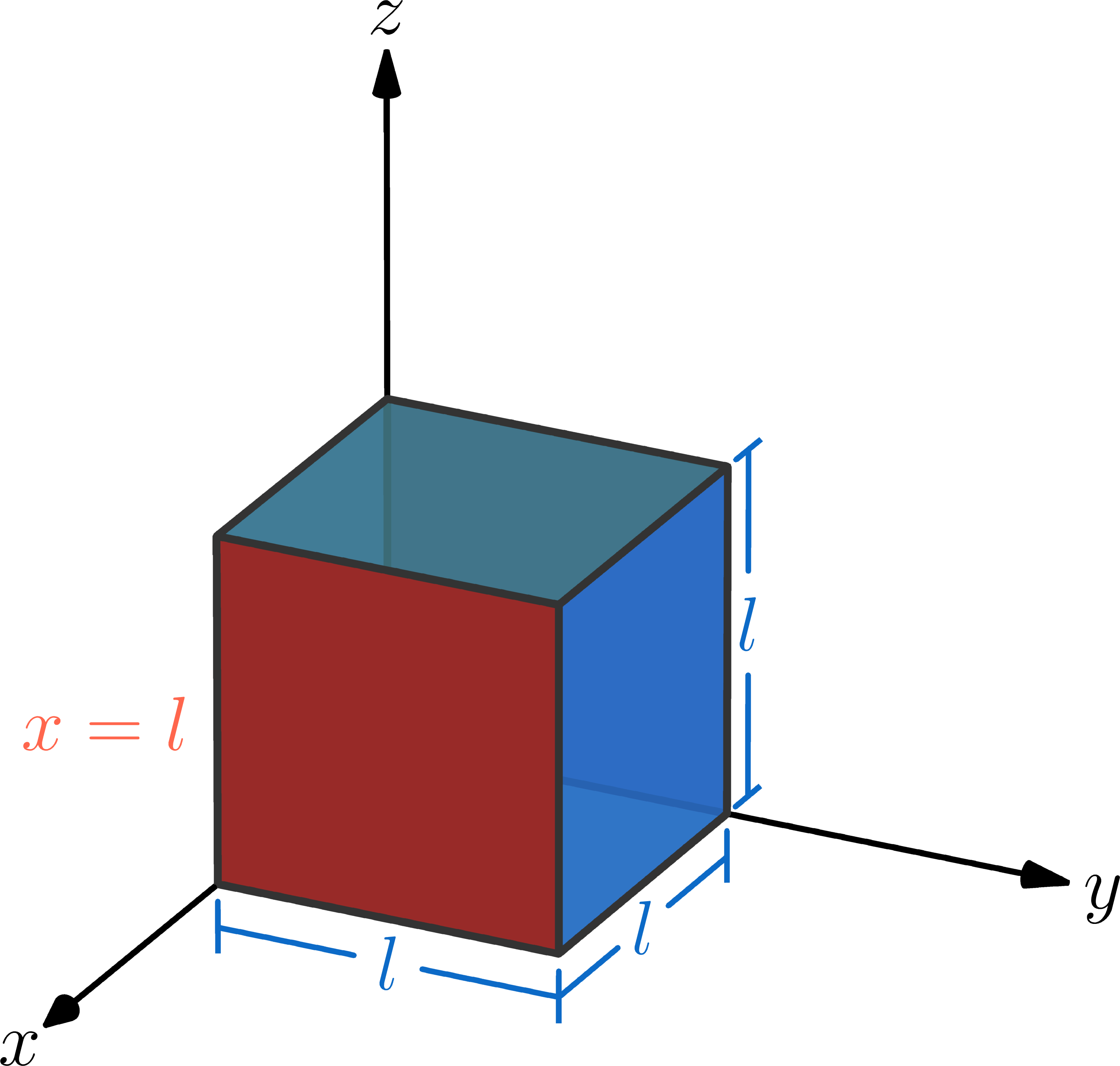The pressure below the surface of a full tank is $p(z) = p_{_\text{fluid}}\left [ 1-\frac{z}{l} \right],$ which is what we saw in the last problem.

Based on this, what double integral does Arthur need to compute for the total force vector on the $x = l$ side of the tank?

# Introducing Surface Integrals

The total force vector on the $x = l$ side of the cubic aquarium tank is given by the integral $\vec{F}_{\text{tot}, x = l} = \left( \iint\limits_{[0,l]\times[0,l]} p_{_\text{fluid}}\left [ 1-\frac{z}{l} \right]dy\, dz \right) \hat{i}.$ Is its magnitude greater than, equal to, or less than that for the bottom of the tank given by $\vec{F}_{\text{bottom}} = - p_{_\text{fluid}} l^2 \hat{k} \ ?$

# Introducing Surface Integrals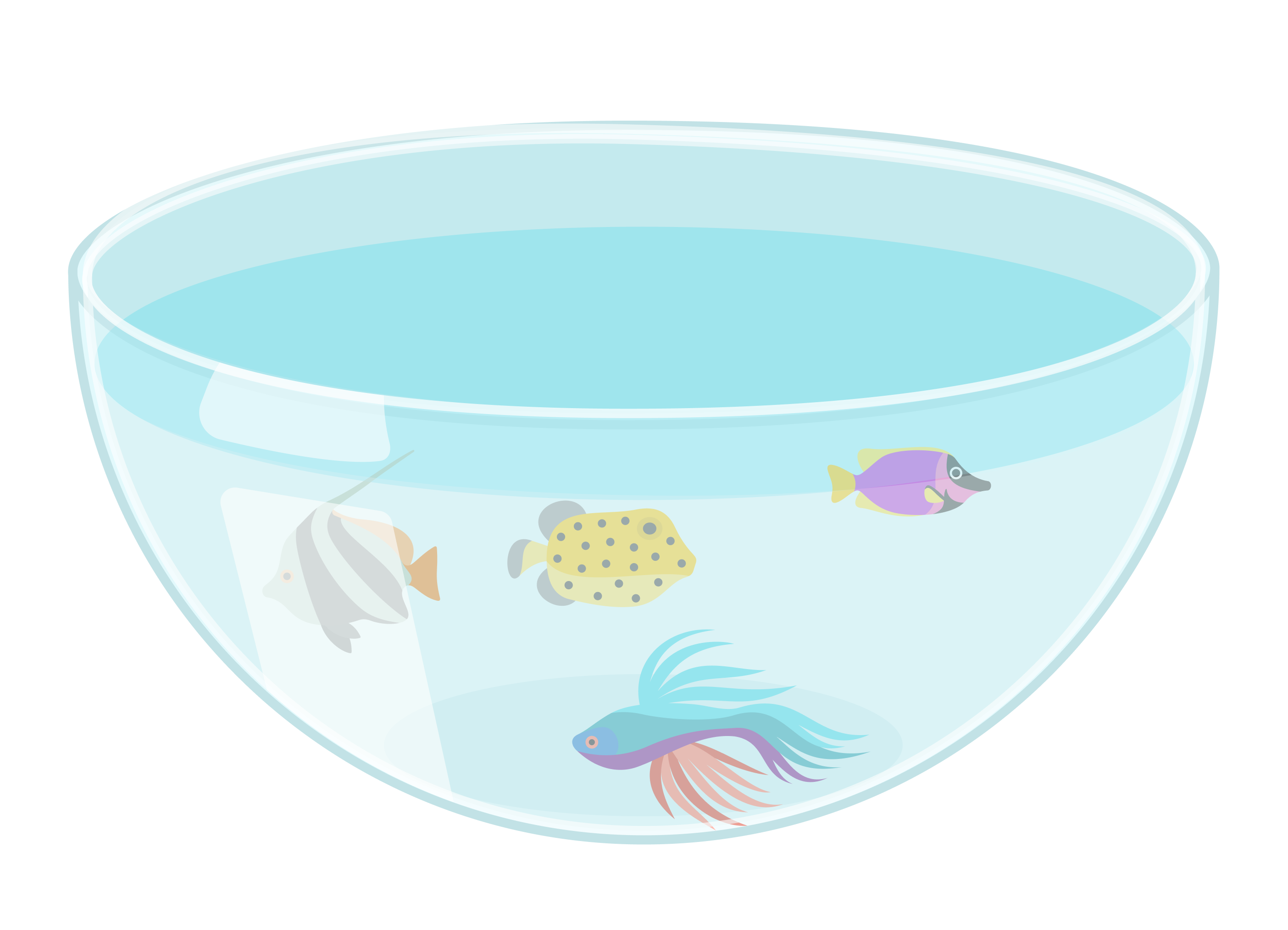Mr. Adams's motto is there's no aquarium shape too weird or too difficult. To initiate Arthur into advanced aquarium design, he's next tasked with constructing a hemispherical tank, a more challenging shape than a cube.

He can approximate the total fluid force just as before, but he'll need to first find the outward-pointing unit normal vector at the point $\left (x,y,l-\sqrt{l^2-x^2-y^2} \right )$ on the hemispherical wall. Here, $l$ is the radius, and the bottom of the bowl sits at $(0,0,0).$

What is this vector?

It's displayed below; sliders $a$ and $b$ control the position of point $\left (a,b, l-\sqrt{l^2-a^2-b^2} \right )$ (all red), and the green arrow represents $\hat{n},$ the outward-pointing unit normal vector there.

# Introducing Surface Integrals

It's still true that the pressure is proportional to depth, and the force is directed perpendicularly outwards even though the wall is now hemispherical.

If Arthur breaks the hemisphere up into very small patches, the total force is approximately $\vec{F}_{\text{tot}} \approx \sum\limits_{i=1}^{n} \sum\limits_{j=1}^{m} p(z_{ij}^{*}) \triangle A_{ij} \hat{n}_{ij} = p_{_\text{fluid}}\sum\limits_{i=1}^{n} \sum\limits_{j=1}^{m} \left [ 1- \frac{z_{ij}^{*}}{l} \right] \triangle A_{ij} \hat{n}_{ij}.$ He knows $\hat{n}_{ij},$ so he just needs to deal with $\triangle A_{ij},$ the area of patch $(i,j).$

The patch (red) sits above a very small rectangle (blue) in the $xy$-plane of dimensions $\triangle x_{i}$ by $\triangle y_j$ and centered on $a=x_{i}^{*},\ b =y_{j}^{*}.$ The Riemann sum for the total fluid force will become a double integral in $x$ and $y$ once $\triangle A_{ij}$ is related to $\triangle x_{i} \triangle y_{j}.$

The picture exaggerates to make things easier to visualize, but the hemispherical patch is approximately a parallelogram with four vertices $\left( x_{i}^{*} \pm \frac{\triangle x_{i}}{2} , \ y_{j}^{*} \pm \frac{\triangle y_{j}}{2}, f \bigg( x_{i}^{*} \pm \frac{\triangle x_{i}}{2} , \ y_{j}^{*} \pm \frac{\triangle y_{j}}{2}\bigg) \right),$ and $f = l - \sqrt{l^2-x^2-y^2}.$ Since $\triangle x_{i}$ and $\triangle y_{j}$ are very, very small, $f \bigg( x_{i}^{*} \pm \frac{\triangle x_{i}}{2} , \ y_{j}^{*} \pm \frac{\triangle y_{j}}{2}\bigg) \approx f \left( x_{i}^{*} , \ y_{j}^{*} \right) \pm \frac{\triangle x_{i}}{2}f_{x}(x_{i}^{*},y_{j}^{*})\pm \frac{\triangle y_{j}}{2}f_{y}(x_{i}^{*},y_{j}^{*}).$ This means that two vectors defining the parallelogram patch are \begin{aligned} \vec{s}_{1} & = \left \langle \triangle x_{i}, 0 , \triangle x_{i} \ f_{x}(x_{i}^{*},y_{j}^{*}) \right \rangle, \ \vec{s}_{2} = \left \langle 0 , \triangle y_{j}, \triangle y_{j} \ f_{y}(x_{i}^{*},y_{j}^{*}) \right \rangle, \end{aligned} and so the area of this parallelogram (i.e. the patch area) is $\triangle A_{ij} \approx \| \vec{s}_{1} \times \vec{s}_{2} \| = \sqrt{1+\big[f_{x}(x_{i}^{*},y_{j}^{*})\big]^2+\big[f_{y}(x_{i}^{*},y_{j}^{*})\big]^2} \triangle x_{i} \triangle y_{j}.$ We'll cover this in greater depth later in our parametric surfaces quiz.

Learn more about first-order approximations and their extensions on our Taylor Series wiki.

# Introducing Surface Integrals

As the size of the patches becomes infinitesimally small, the total force Riemann sum approaches the double integral \begin{aligned} \vec{F}_{\text{tot}} & = p_{_\text{fluid}} \iint\limits_{x^2+y^2 \leq l^2} \left [ 1-\frac{f(x,y)}{l} \right] \hat{n}\, d A, \end{aligned} where we abbreviate $d A = \sqrt{ 1+[f_{x}]^2+[f_{y}]^2}\, dx\, dy$ and $f(x,y) = l - \sqrt{l^2-x^2-y^2},\ \ \hat{n} =\left \langle \frac{x}{l} , \frac{y}{l} , \frac{f(x,y)}{l}-1 \right \rangle.$ What's the $\hat{i}$-component of the total fluid force?

Hint: Think about the symmetry of the problem to reduce the amount of integration needed.

# Introducing Surface Integrals

So the only nonzero component of the total fluid force is in the $\hat{k}$-direction: $\vec{F}_{\text{tot}} = -p_{_\text{fluid}} \left( \iint\limits_{x^2+y^2\leq l^2} \sqrt{1-\left( \frac{x^2+y^2}{l^2} \right)}\ dx\, dy \right) \hat{k}.$ Use polar coordinates $x = r \cos(\theta), \ y = r \sin(\theta)$ and the identity $\int\limits_{u=0}^{u=1} u \sqrt{1-u^2}\, du = \frac{1}{3}$ to find the total fluid force on the hemispherical tank that Arthur needs for his design.

# Introducing Surface Integrals

Arthur's well on his way to becoming a master builder thanks to a little vector calculus!

Finding the total fluid force on the hemispherical tank $S$ amounted to computing $\vec{F}_{\text{tot}} = \iint_{S} p \ \hat{n}\, dA,$ a new kind of integral over a surface, or surface integral.

Since they're an essential part of vector calculus, we'll have a whole series of quizzes devoted just to surface integrals.

In the next quiz, we'll use what we learned here to begin to understand one of the deepest connections in all of vector calculus: the relation between surface integrals and divergence.

# Introducing Surface Integrals

×